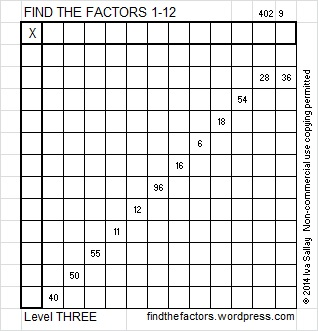# 25 and Level 3

25 is a composite number, and it is 5 squared. 25 = 1 x 25 or 5 x 5. Factors of 25: 1, 5, 25. Prime factorization: 25 = 5 x 5, which can also be written 25 = 5².

Since √25 = 5, a whole number, 25 is a perfect square.When 25 is a clue in the FIND THE FACTORS puzzles, write 5 in both the corresponding factor row and the factor column.Write the numbers 1-12 in the top row and again in the first column so that those numbers are the factors of the given clues. Use logic, not guessing and checking, to find its unique solution. Level 3 puzzles are designed to be solved starting from a row at the top of the puzzle with 2 clues. First find the common factor of those two clues that will allow you to write only numbers from 1 to 12 in the factor row at the top of the puzzle. Then factor row by row to the bottom of the puzzle making sure each number from 1 to 12 is written only once in both the factor row and the factor column. You will notice a rhythm for the answers as you work. Good luck!

This week’s puzzles are also available in an excel file here if you have a spreadsheet program on your computer. If you enable editing in excel, you can type your answers directly onto the puzzle, and you can also easily print the puzzles.

Here is the solution to last week’s level 3 puzzle:This site uses Akismet to reduce spam. Learn how your comment data is processed.